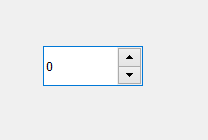# PyQt5 QSpinBox Widget

• Last Updated : 06 May, 2020

QSpinBox is a PyQt5 widget which presents the user with a textbox that displays an integer with up/down button on its right. The value in the textbox increases/decreases if the up/down button is pressed. The default minimum value is 0 and maximum value is 99.Example :
A window having a Spinbox, when value changes a message will appear displaying the current value.

Steps for implementation –

1. Create main window class
2. Create a spin box widget
3. Create a label to show the value
4. Add action to the spin box such that when value is changed action should get called
5. Inside the action set value to the label

Below is the implementation

 `# importing libraries ` `from` `PyQt5.QtWidgets ``import` `*`  `from` `PyQt5 ``import` `QtCore, QtGui ` `from` `PyQt5.QtGui ``import` `*`  `from` `PyQt5.QtCore ``import` `*`  `import` `sys ` ` `  ` `  `class` `Window(QMainWindow): ` ` `  `    ``def` `__init__(``self``): ` `        ``super``().__init__() ` ` `  `        ``# setting title ` `        ``self``.setWindowTitle(``"Python "``) ` ` `  `        ``# setting geometry ` `        ``self``.setGeometry(``100``, ``100``, ``600``, ``400``) ` ` `  `        ``# calling method ` `        ``self``.UiComponents() ` ` `  `        ``# showing all the widgets ` `        ``self``.show() ` ` `  `    ``# method for widgets ` `    ``def` `UiComponents(``self``): ` ` `  `        ``# creating spin box ` `        ``self``.spin ``=` `QSpinBox(``self``) ` ` `  `        ``# setting geometry to spin box ` `        ``self``.spin.setGeometry(``100``, ``100``, ``100``, ``40``) ` ` `  `        ``# adding action to the spin box ` `        ``self``.spin.valueChanged.connect(``self``.show_result) ` ` `  `        ``# creating label show result ` `        ``self``.label ``=` `QLabel(``self``) ` ` `  `        ``# setting geometry ` `        ``self``.label.setGeometry(``100``, ``200``, ``200``, ``40``) ` ` `  `    ``# method called by spin box ` `    ``def` `show_result(``self``): ` ` `  `        ``# setting value of spin box to the label ` `        ``self``.label.setText(``"Value : "` `+` `str``(``self``.spin.value())) ` ` `  ` `  `# create pyqt5 app ` `App ``=` `QApplication(sys.argv) ` ` `  `# create the instance of our Window ` `window ``=` `Window() ` ` `  `window.show() ` ` `  `# start the app ` `sys.exit(App.``exec``()) `

Output :

My Personal Notes arrow_drop_up
Recommended Articles
Page :